# NEET Physics: NEET Physics Questions, Study Materials, Paper Analysis

## NEET Physics: NEET Physics Questions, Study Materials, Paper Analysis

### Notes and Study Materials -Gravitation

Kepler’s laws of planetary motion. The universal law of gravitation. Acceleration due to gravity and its variation with altitude and depth. Gravitational potential energy; gravitational potential. Escape velocity, orbital velocity of a satellite. Geostationary satellites.

### NEWTON’S UNIVERSAL LAW OF GRAVITATION

Gravitational force is an attractive force between any two point masses M1 and M2 separated by any distance r.
It is given by F = Gwhere G is the universal gravitational constant.
Its value, G = 6.67 × 10–11 Nm2 kg–2
Dimensions of G are [M–1 L3 T–2]
• The gravitational force is the central force and follows inverse square law. It acts along the line joining the particles.
• Since the work done by the gravitational force is independent of the path followed and hence it is a conservative force.
• It is the weakest force in nature. It is 1038 times smaller than nuclear force and 1036 times smaller than electric force. Strongest force being nuclear force (for small range) followed by electric force.
• Gravitation is independent of the presence of other bodies around it.

### ACCELERATION DUE TO GRAVITY (g)

The force of attraction exerted by earth on a body of mass m is the force of gravity.
So the force of gravity from Newton’s gravitational law is……(i)
where   Me = mass of earth
r = distance of the body from the centre of earth.
The force of gravity can be written as F = mg ……(ii)
where g is called the acceleration due to gravity.
From the expression (i) and (ii), we get……(iii)
If body is located at the surface of earth i.e., r = Re (radius of earth) then……(iv)
where ρ is the density of earth.
This is the relation between universal gravitational constant ‘G’ and acceleration due to gravity ‘g’.

#### CHANGE IN THE VALUE OF ACCELERATION DUE TO GRAVITY (g)

• Due to rotation or latitude of earth
Let us consider a particle P at rest on the surface of earth at a latitude φ.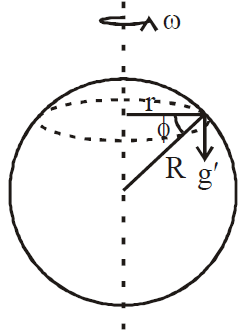At poles φ = 90º, so g(φ) = g …(v)
At equator φ = 0º so g(φ) = g –ω2Re …(vi)
• Due to shape of earth
The shape of the earth is not perfectly spherical. It is flattened at poles. The polar radius is 21 km less than the equatorial radius. Hence acceleration due to gravity at poles is greater than at the equator.
gp = 9.83 ms–2 = value of g at poles
ge = 9.78 ms–2 = values of g at equator
i.e. gp > ge
• At a depth ‘d’ below the earth surface
The acceleration due to gravity at the surface of earth.(from eqn. iv) …(vii)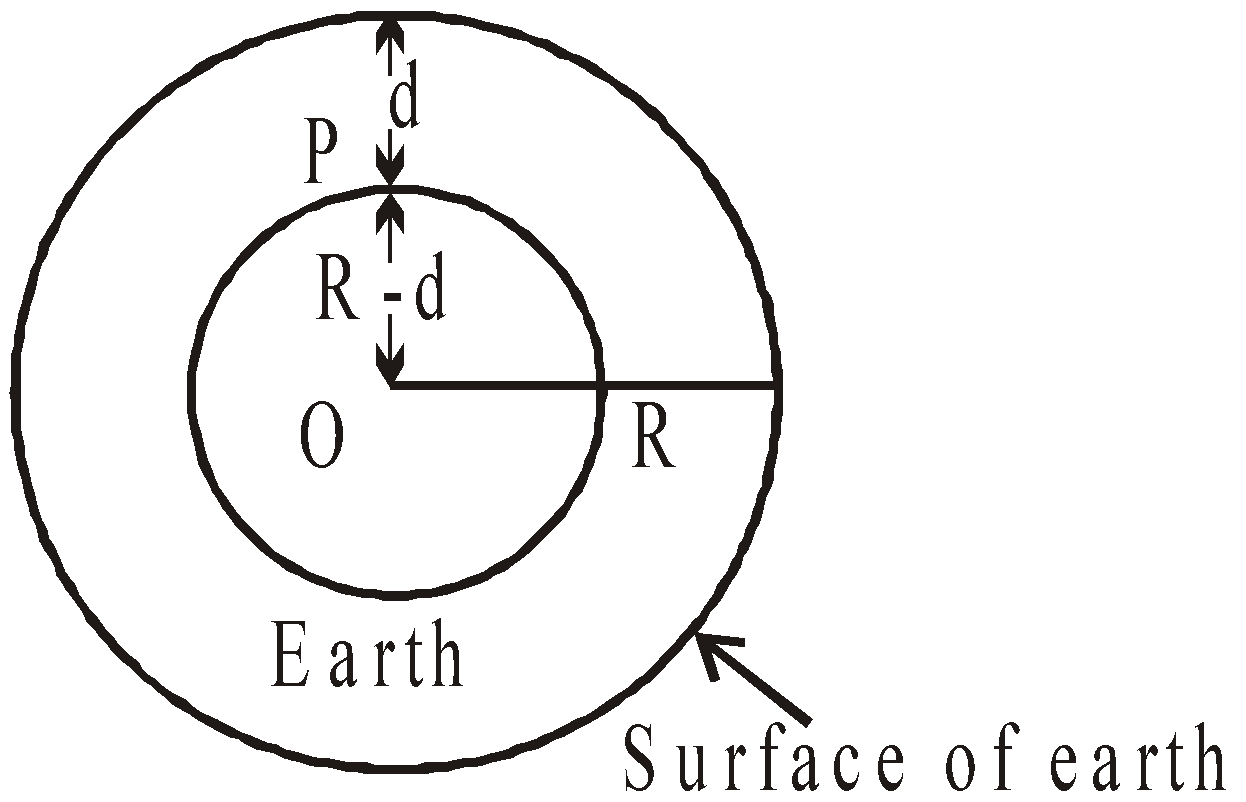If a body is taken at a depth d below the earth surface, then the body is attracted by inner core of mass M’ of earth radius (Re– d). Then acceleration due to gravity at point P is…(viii)
From eqns. (vii) and (viii) we have…(ix)
The value of acceleration due to gravity at the centre of the earth is zero.
• At a height ‘h’ above the earth surface
The acceleration due to gravity at the surface of earth from expression (iv) is defined asAt a height h above the earth surface, the acceleration due to gravity is…(x)[from eqs. (ix) & (x)]
If h << Re…(xi)
KEEP IN MEMORY
1. The value of the acceleration due to gravity on the moon is about one sixth of that on the earth and on the sun is about 27 times that on the earth.1. The value of g is minimum on the mercury, among all planets.
2. For h<<R, the rate of decrease of the acceleration due to gravity with height is twice as compared to that with depth.
3. The value of g increases with the increase in latitude. Its value at latitude θ is given by : gθ= g – Rω2 cos2θ.
4. Rotation of the earth about its own axis is responsible for decrease in the value of g with latitude.
5. The weight of the body varies along with the value of g (i.e. W = mg)
6. Inertial mass and gravitational mass
1. Inertial mass (Mi) defined by Newton’s law of motion
Mi = F/a =(iff applied force on (Mi)1 and (Mi)2 is same.)
1. Gravitational mass Mg defined by Newton’s law of gravitation
Mg =1. If two spheres of same material, mass and radius are put in contact, the gravitational attraction between them is directly proportional to the fourth power of the radius.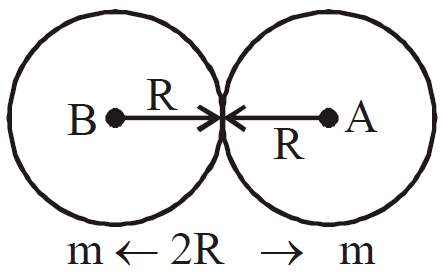This system may be considered to be made up of two mass each separated by 2R distance.
1. If the earth stops rotating about its axis, the value of g at the equator will increase by about 0.35%, but that at the poles will remain unchanged.
If the earth starts rotating at the angular speed of about 17 times the present value, there will be weightlessness on equator, but g at the poles will remain unchanged. In such a case, the duration of the day will be about 84 min.
1. If the radius of planet decreases by n%, keeping the mass unchanged, the acceleration due to gravity on its surface increases by 2n%. i.e.,2. If the mass of the planet increases by m% keeping the radius constant, the acceleration due to gravity on its surface increases by m% i.e.,where R = constant.
3. If the density of planet decreases by ρ% keeping the radius constant, the acceleration due to gravity decreases by ρ%.
4. If the radius of the planet decreases by r% keeping the density constant, the acceleration due to gravity decreases by r%.

### GRAVITATIONAL FIELD

(or Gravitational Field Intensity)
The region or space around a body in which its gravitational influence is experienced by other bodies is the gravitational field of that body.
The gravitational field strength Eg, produced by a mass M at any point P is defined as the force exerted on the unit mass placed at that point P. Then…(i)
where m = test mass
r = distance between M and m
The direction of Eg always points towards the mass producing it.
The gravitational field can be represented by gravitational lines of force.
The S.I unit of Eg is newton/kg. Its dimensions are [M0LT–2].
GRAVITATIONAL INTENSITY (Eg) FOR SPHERICAL SHELL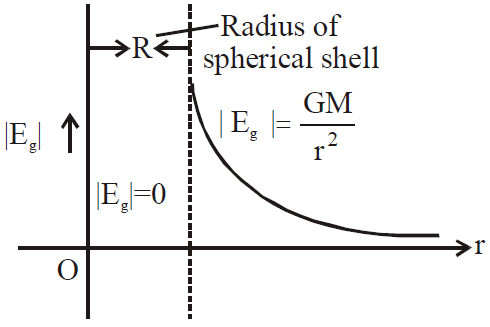• |Eg|= 0 at points inside the spherical shell (i.e. r < R)
•at the surface of shell (i.e. r = R)
•for outside the spherical shell (r >R)
It is clear that as r increases, Eg decreases.
GRAVITATIONAL INTENSITY FOR SOLID SPHERE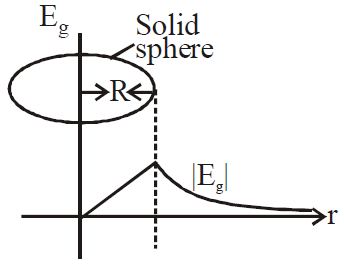•for points inside the solid sphere (r<R)
•At the surface of solid sphere (r = R)
•Outside the solid sphere (r > R)

### GRAVITATIONAL POTENTIAL

Gravitational potential at any point is the work done by gravitational force in carrying a body of unit mass from infinity to that point in gravitational influence of source. i.e.,where r is the distance of point from source mass M.
The S.I. unit of V is joule/kg. Its dimensions are [M0L2T–2]. It is a scalar quantity.
GRAVITATIONAL POTENTIAL AT THE EARTH SURFACEGRAVITATIONAL POTENTIAL DUE TO A UNIFORM RING AT A POINT ON ITS AXIS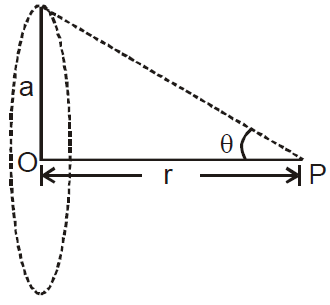GRAVITATIONAL POTENTIAL DUE TO A UNIFORM THIN SPHERICAL SHELL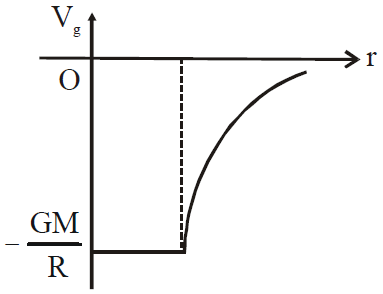• at a point outside the shell,(r > R)
• at a point inside the shell,(r < R)
where R = radius of spherical shell.
Potential due to a uniform spherical shell is constant throughout the cavity of the shell.
GRAVITATIONAL POTENTIAL DUE TO A UNIFORM SOLID SPHERE
• at an external point(r≥ R)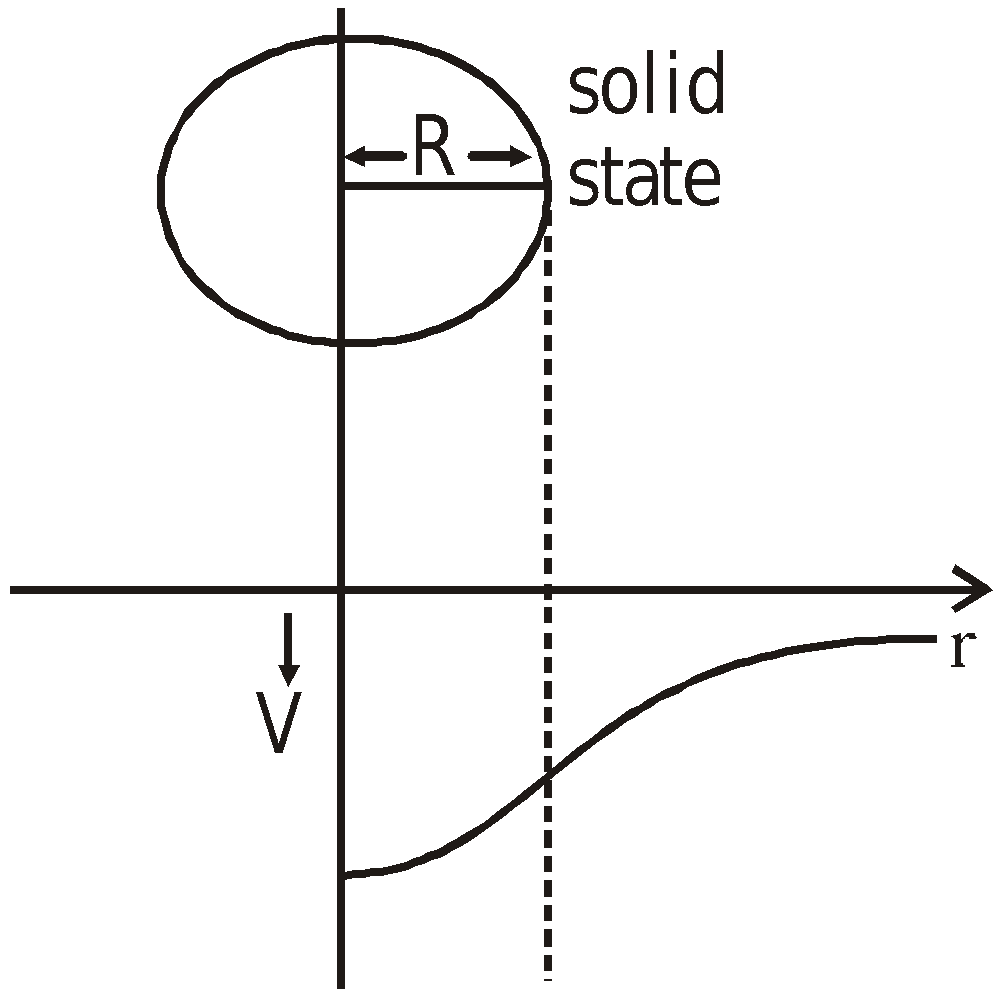• at an internal point(r < R)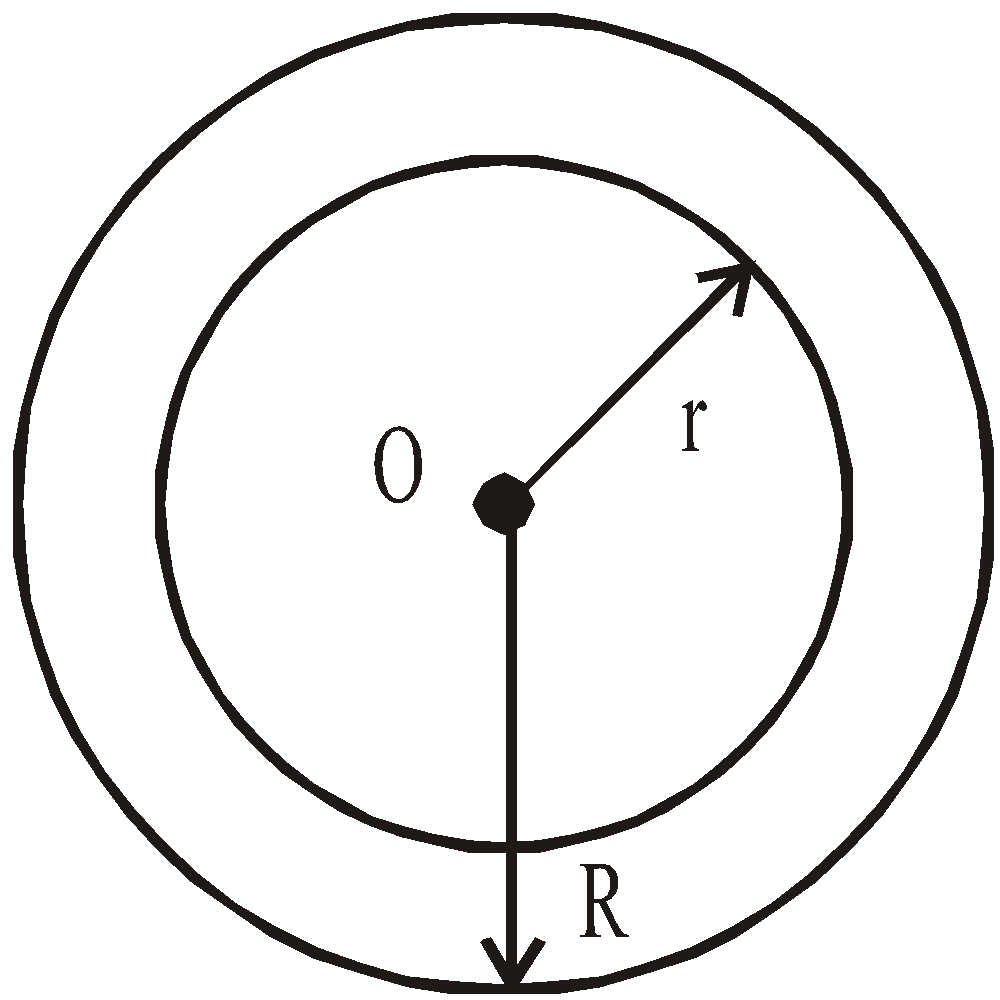• Potential at the centre of solid sphere is### GRAVITATIONAL POTENTIAL ENERGY

Let Vg be the gravitational potential at a point. If we place a mass m at that point, then we say that the gravitational potential energy possessed by the mass isWe can also therefore say that the gravitational potential energy of a system containing two masses m1 and m2 placed such that the centre to centre distance between them is r thenThis formula is valid taking into consideration the fact that we have taken the gravitational potential of few at infinity. Please remember that unless otherwise stated it is understood that the gravitational potential is taken to be zero at infinity.
Relation between gravitational potential energy and gravitational potential
Gravitational potential energy = gravitational potential × mass
KEEP IN MEMORY
1. The gravitational potential energy of a mass m at a point above the surface of the earth at a height h is given by. The –ve sign shows that if h increases, the gravitational PE decreases and becomes zero at infinity.
2. If we take reference level to be at the surface of earth (not at infinity) i.e., we assume that the gravitational P.E of a mass m is zero at the surface of earth, then the gravitational potential energy at a height h above the surface of earth is (mgh), where h << Re (radius of earth)
3. The gravitational P.E of mass m on the earth’s surface isIf we assume that gravitational P.E of mass m is zero at infinity i.e., we take reference level at infinity then P.E.of any mass m at a height h above the earth surface
So work done against the gravitational force, when the particle is taken from surface of earth to a height h above the earth surface
Work done = change in potential energy
⇒ Uh–Ug =(if h<<R)So, work done = mgh is stored in the particle as particle potential energy, when kept at height ‘h’.

### SATELLITES

A satellite is any body revolving around a large body under the gravitational influence of the latter.
The period of motion T of an artificial satellite of earth at a distance h above the surface of the earth is given by,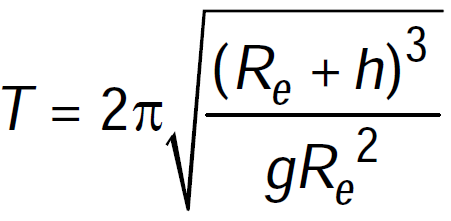where, Re = radius of the earth
where,    g = acceleration due to gravity on the surface of the earth.
If Re > > h, then;  Re = 6.4 × 106 m ; g = 9.8 ms–2
i.e.Assuming Re + h = r, the distance of the satellite from the centre of the earth,

#### ORBITAL VELOCITY (V0 )

Let a satellite of mass m revolve around the earth in circular orbit of radius r with speed v0. The gravitational pull between satellite and earth provides the necessary centripetal force.
Centripetal force required for the motion =Gravitational force =oror………. (1)
or[∵and r = (R + h)]
Orbital velocity,• Value of orbital velocity does not depend on the mass of the satellite.
• Around the earth the value of orbital velocity is 7.92 km/sec.
• Greater is the height of the satellite, smaller is the orbital velocity.
• The direction of orbital velocity is along the tangent to the path.
• The work done by the satellite in a complete orbit is zero.

#### ANGULAR MOMENTUM (L)

For satellite motion, angular momentum will be given byi.e., L = [m2GMr]1/2
Angular momentum of a satellite depends on both, the mass of orbiting and central body. It also depends on the radius of the orbit.

#### ENERGY OF A SATELLITE

Total energy of satellite revolving in an orbit of radius r around the earth can be calculated as follows :
• The gravitational potential energy of a satellite of mass m, where r is the radius of the orbit.
• Kinetic energy of the satellite• So, total energy of the satelliteThe negative sign shows that satellite cannot leave the orbit itself. It requires an energy equal to, which is called Binding Energy (B.E.) of satellite.
• Total energy of a satellite at a height equal to the radius of the earth#### GEO-STATIONARY SATELLITE

A satellite which appears to be stationary for a person on the surface of the earth is called geostationary satellite.
It is also known as parking satellite or synchronous satellite.
• The orbit of the satellite must be circular and in the equatorial plane of the earth.
• The angular velocity of the satellite must be in the same direction as the angular velocity of rotation of the earth i.e., from west to east.
• The period of revolution of the satellite must be equal to the period of rotation of earth about its axis. i.e. 24 hours = 24 × 60 × 60 = 86400 sec.or== 42237 × 103 m = 42,237 km. ≈ 42000 km.
h = r – R = 42000 – 6400 = 35600 km.
• Height of geostationary satellite from the surface of the earth is nearly 35600 km.
• The orbital velocity of this satellite is nearly 3.08 km/sec.
• The relative velocity of geostationary satellite with respect to earth is zero.
• This type of satellite is used for communication purposes. The orbit of a geostationary satellite is called ‘Parking Orbit’.

#### POLAR SATELLITE

Polar satellites travel around the earth in an orbit that travels around the earth over the poles. The earth rotates on its axis as the satellite goes around the earth. Thus over a period of many orbits it looks down on every part of the earth.
Different orbital shapes corresponding to different velocities of a satellite :
• When v < v0
• The path is spiral. The satellite finally falls on the earth
• Kinetic energy is less than potential energy
• Total energy is negative
• When v = v0
• The path is circular
• Eccentricity is zero
• Kinetic energy is less than potential energy
• Total energy is negative
• When v0 < v < ve
• The path is elliptical
• Eccentricity < 1
• Kinetic energy is less than potential energy
• Total energy is negative
• When v = ve
• The path is a parabola
• Eccentricity = 1
• Kinetic energy is equal to potential energy
• Total energy is zero
• When v > ve
• The path is a hyperbola
• Eccentricity > 1
• Kinetic energy is greater than potential energy
• Total energy is positive
If the orbit of a satellite is elliptical
• The energy= const. with ‘a’ as semi-major axis;
• KE will be maximum when the satellite is closest to the central body (at perigee) and minimum when it is farthest from the central body (at apogee) [as for a given orbit L = const.,
i.e., mvr = const., i.e., v ∝ 1/r]
• PE = (E – KE) will be minimum when KE = max, i.e., the satellite is closest to the central body (at perigee) and maximum when KE = min, i.e., the satellite is farthest from the central body (at apogee).

### ESCAPE SPEED (Ve)

It is the minimum speed with which a body should be projected from the surface of a planet so as to reach at infinity i.e., beyond the gravitational field of the planet.
If a body of mass m is projected with speed v from the surface of a planet of mass M and radius R, then
asand, ES =Now if v’ is the speed of body at ∞, then[as]
So by conservation of energyi.e.so v will be minimum when v′ → 0,
i.e.• The value of escape velocity does not depend upon the mass of the projected body, instead it depends on the mass and radius of the planet from which it is being projected.
• The value of escape velocity does not depend on the angle and direction of projection.
• The value of escape velocity from the surface of the earth is 11.2 km/sec.
• The minimum energy needed for escape is = GMm/R.
• If the velocity of a satellite orbiting near the surface of the earth is increased by 41.4%, then it will escape away from the gravitational field of the earth.
• If a body falls freely from infinite distance, then it will reach the surface of earth with a velocity of 11.2 km/sec.
Relation between orbital velocity (V0) and escape speed (Ve)KEEP IN MEMORY
1. The escape velocity on moon is low as (as gm=gE/6) hence there is no atmosphere on moon.
2. If the orbital radius of the earth around the sun be one fourth of the present value, then the duration of the year will be one eighth of the present value.
3. The satellites revolve around the earth in a plane that coincides with the great circle around the earth.

### KEPLER’S LAWS OF PLANETARY MOTION

#### LAW OF ORBITS

Each planet revolves about the sun in an elliptical orbit with the sun at one of the foci of the ellipse.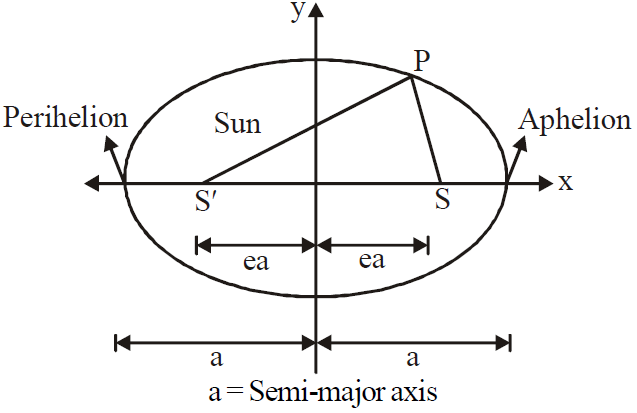A planet of mass m moving in an elliptical orbit around the sun. The sun of mass M, is at one focus S′ of the ellipse. The other focus is S, which is located in empty space. Each focus is at distance ‘ea’ from the ellipse’s centre, with ‘e’ being the eccentricity of the ellipse and ‘a’ semimajor axis of the ellipse, the perihelion (nearest to the sun) distance rmin., and the aphelion (farthest from the sun) distance rmax. are also shown.
rmax = a + e a = (1 + e) a
rmin = a – e a = (1 – e) a
The distance of each focus from the centre of ellipse is ea, where e is the dimensionless number between 0 to 1 called the eccentricity. If e = 0, the ellipse is a circle.
For earth e = 0.017.

#### LAW OF AREAS

An imaginary line that connects a planet to the sun sweeps out equal areas in the plane of the planet’s orbit in equal times.
i.e., the rate dA/dt at which it sweeps out area A is constant.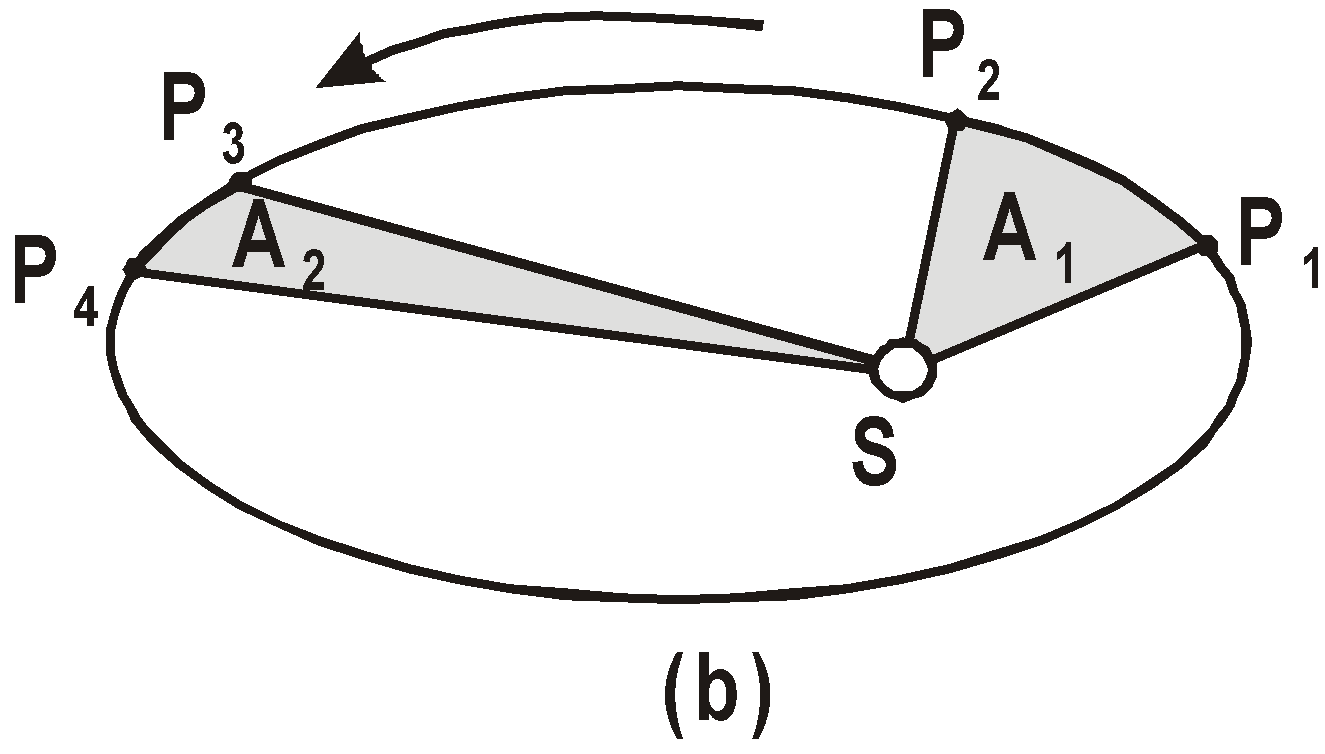Ifthen A1 = A2and as L = mvr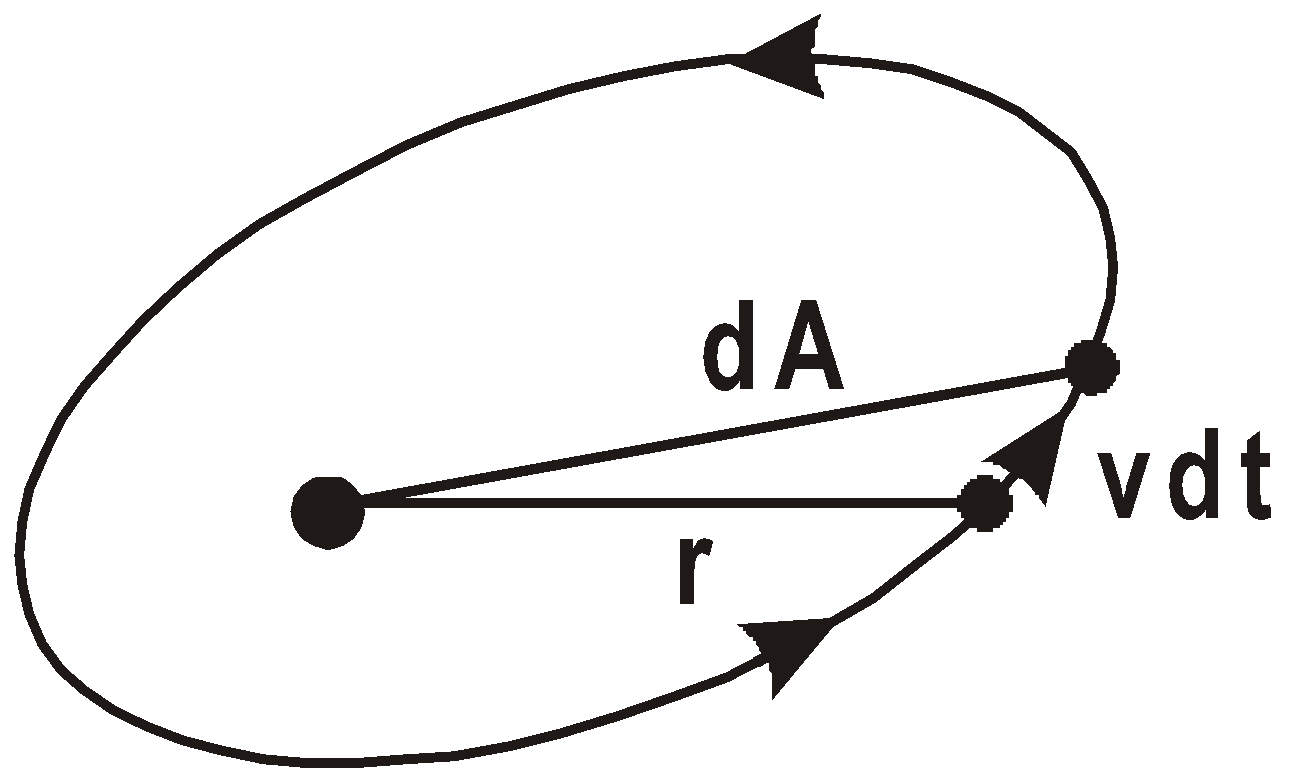so….(1)
But as L = constt., (force is central, so torque = 0 and hence angular momentum of the planet is conserved)
Areal velocity (dA/dt) = constant which is Kepler’s IInd law, i.e., Kepler’s IInd law or constancy of areal velocity is a consequence of conservation of angular momentum.

#### LAW OF PERIODS

The square of the period of revolution of any planet is proportional to the cube of the semi-major axis of the orbit.
i.e.,   T2 ∝ r3.
or,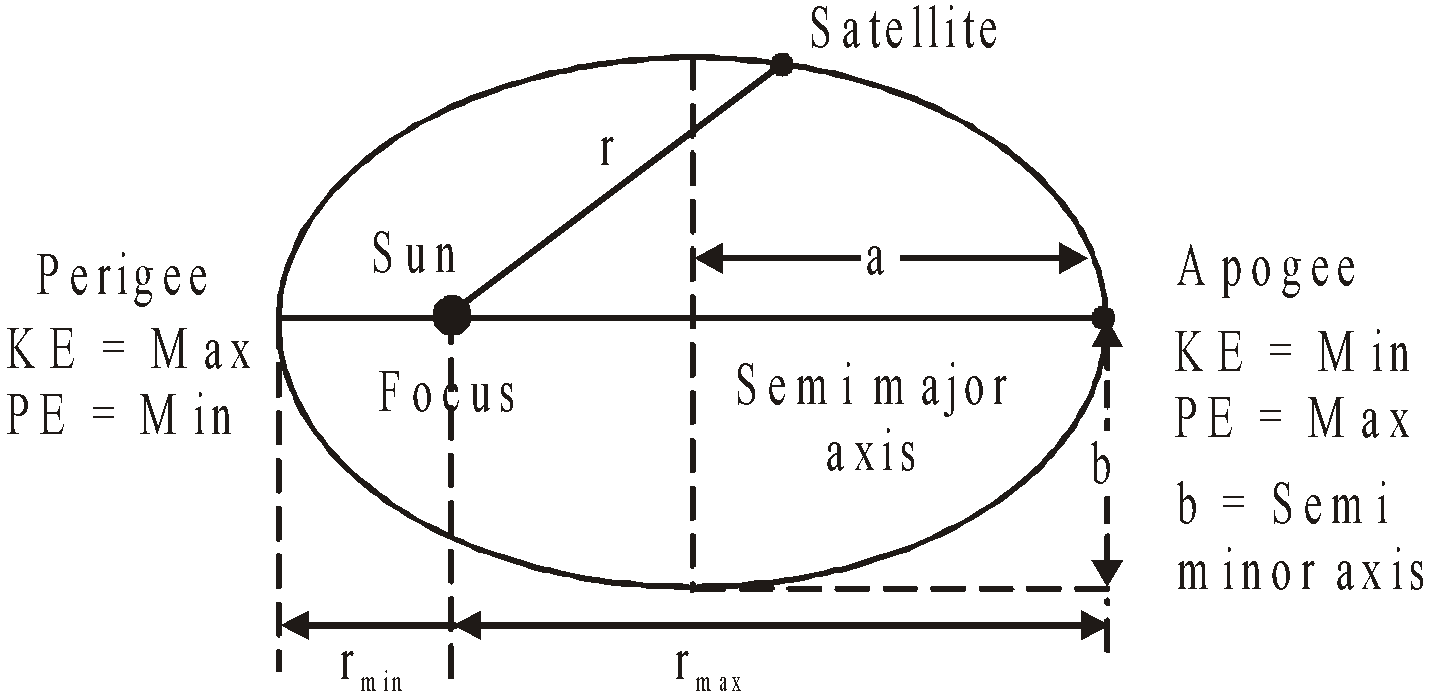### WEIGHTLESSNESS

The “weightlessness” you may feel in an aircraft occurs any time the aircraft is accelerating downward with acceleration g. It is possible to experience weightlessness for a considerable length of time by turning the nose of the craft upward and cutting power so that it travels in a ballistic trajectory. A ballistic trajectory is the common type of trajectory you get by throwing a rock or a baseball, neglecting air friction. At every point on the trajectory, the acceleration is equal to g downward since there is no support. A considerable amount of experimentation has been done with such ballistic trajectories to practice for orbital missions where you experience weightlessness all the time.
The satellite is moving in a circular orbit, it has a radial accelerationi.e., it is falling towards earth’s centre with acceleration a,
so apparent weight of the body in it Wap = m(g´ – a)
where g´ is the acceleration due to gravity of earth at the position (height) of satellite, i.e. g´ = (GM/r2), so thati.e., the apparent weight of a body in a satellite is zero and is independent of the radius of the orbit.
KEEP IN MEMORY
1. The moon takes 27.3 days to revolve around the earth.
The radius of its orbit is 3.85 × 105 km.
2. Kepler’s second law is based on conservation of angular momentum.
3. Perihelion distance is the shortest distance between the sun and the planet.
4. Aphelion distance is the largest distance between the Sun and the planet.1. If e is the eccentricity of the orbit
thenraphelion + rperihelion = 2r
1. If e > 1 and total energy (KE + PE) > 0, the path of the satellite is hyperbolic and it escapes from its orbit.
2. If e < 1 and total energy is negative it moves in elliptical path.
3. If e = 0 and total energy is negative it moves in circular path.
4. If e = 0 and total energy is zero it will take parabolic path.
5. The path of the projectiles thrown to lower heights is parabolic and thrown to greater heights is elliptical.
6. Kepler’s laws may be applied to natural and artificial satellites as well.
7. Gravitational force does not depend upon medium so no medium can shield it or block it.
8. The escape velocity and the orbital velocity are independent of the mass of the body being escaped or put into the orbit.